Checkout JEE MAINS 2022 Question Paper Analysis : Checkout JEE MAINS 2022 Question Paper Analysis :

# Angle Relationships: Parallel Lines & Transversal

In geometry, lines are classified into several types such as parallel, perpendicular, intersecting and non-intersecting lines, etc. For non-intersecting lines, we can draw a special line called transversal that intersects these lines at different points. In this article, you will learn about parallel lines, transversals and angle relationships with these lines along with suitable images and examples.

### Parallel lines

Two lines are said to be parallel when they do not intersect each other. We can also say that two lines that run along and meet at infinity are called parallel lines.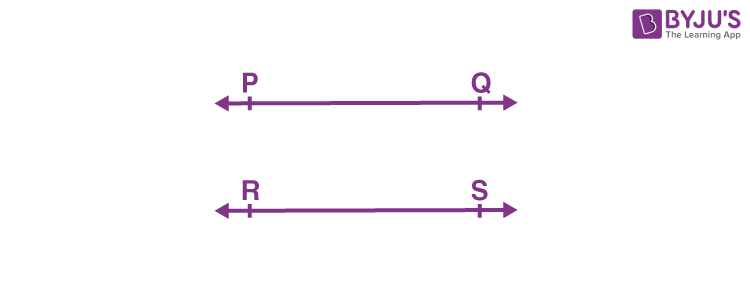### Transversals

When a line intersects two lines at distinct points, it is called a transversal. In the below figure, line l intersects a and b at two distinct points, P and Q. Therefore, line l is the transversal line.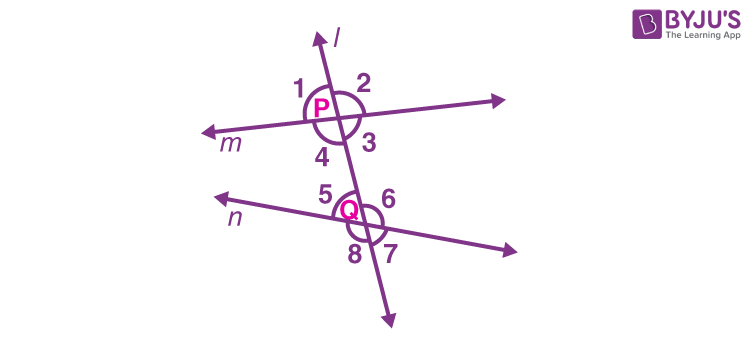In the figure below, the line EF is not a transversal even if it intersects two lines AB and CD at O because it does not cut both lines at two distinct points. For a line to be transversal, it must cross two or more lines at separate points.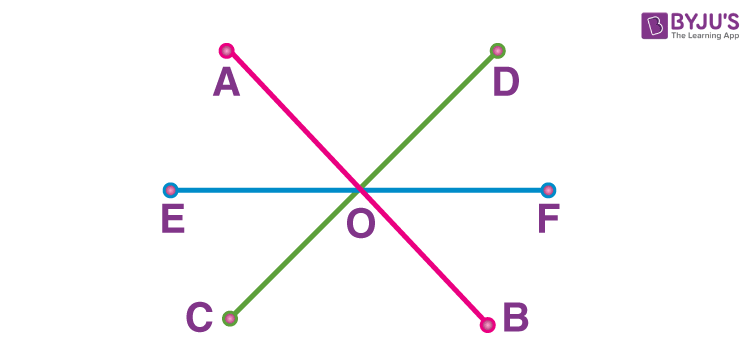## Angle Relationship Between the Parallel Lines and Transversal

Various angle pairs are formed when a transversal intersects two or more parallel lines. Let us quickly recapitulate the angle relationships for the parallel lines cut by a transversal. Let a and d be two parallel lines intersected by the transversal l at the points P and Q, as shown in the figure below.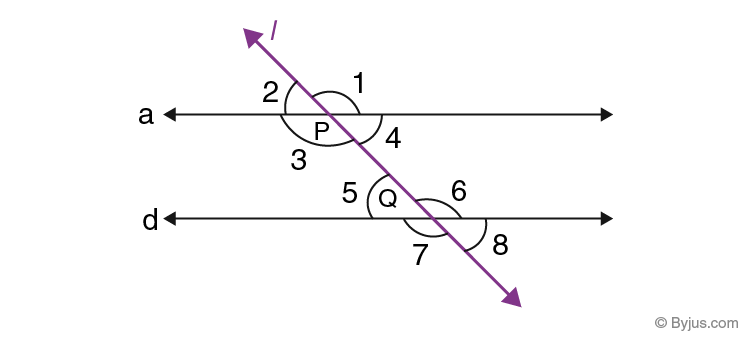Now, we can write different pairs of angles and their corresponding relationships.

Corresponding Angles:

Corresponding angles are the angles which are formed in matching corners or corresponding corners with the transversal when two parallel lines and the transversal.

∠1 and ∠6

∠4 and ∠8

∠2 and ∠5

∠3 and ∠7

Also, if a transversal intersects two parallel lines, then each pair of corresponding angles is equal. Thus,

∠1 = ∠6

∠4 = ∠8

∠2 = ∠5

∠3 = ∠7

Alternate interior angles:

Alternate interior angles are the angles formed when a transversal intersects two coplanar lines.

∠4 and ∠5

∠3 and ∠6

However, if a transversal intersects two parallel lines, then each pair of alternate interior angles is equal.

∠4 = ∠5

∠3 = ∠6

Alternate exterior angles:

Alternate exterior angles are the pair of angles that are formed on the outer side of two lines but on the opposite side of the transversal.

∠1 and ∠7

∠2 and ∠8

If two parallel lines are cut by a transversal, then the resulting alternate exterior angles are congruent.

∠1 = ∠7

∠2 = ∠8

Interior angles on the same side of the transversal:

Interior angles on the same side of the transversal are also referred to as consecutive interior angles or allied angles or co-interior angles.

∠3 and ∠5

∠4 and ∠6

If a transversal intersects two parallel lines, then each pair of interior angles on the same side of the transversal is supplementary, i.e. they add up to 180°.

∠3 + ∠5 = 180°

∠4 + ∠6 = 180°

Vertically opposite angles

When two lines intersect each other, then the opposite angles, formed due to intersection, are called vertical angles or vertically opposite angles. Also, a pair of vertical angles are always congruent.

So,

∠1 = ∠3

∠2 = ∠4

∠7 = ∠6

∠8 = ∠5

The summary of angle relationships of parallel lines and transversal as given in the above figure is tabulated below:

 Pair of angles formed Angle relationships Pairs of Corresponding angles ∠1 = ∠6, ∠4 = ∠8, ∠2 = ∠5, ∠3 = ∠7 Pairs of Alternate interior angles ∠4 = ∠5, ∠3 = ∠6 Pairs of Alternate exterior angles ∠1 = ∠7, ∠2 = ∠8 Pairs of Interior angles on the same side of the transversal ∠3 + ∠5 = 180°, ∠4 + ∠6 = 180° Pairs of Vertically opposite angles ∠1 = ∠3, ∠2 = ∠4, ∠7 = ∠6, ∠8 = ∠5

Now, the question arises: how can we deduce if two lines are parallel or not? Is there any condition that demonstrates the parallel nature of two or more lines? Consider this situation.

His teacher asked Alan to draw two parallel lines. With the help of his set squares and ruler, he drew a straight line segment AB and then placed the set square on this line and drew two line segments XY and PQ, by changing the position of the set squares as shown.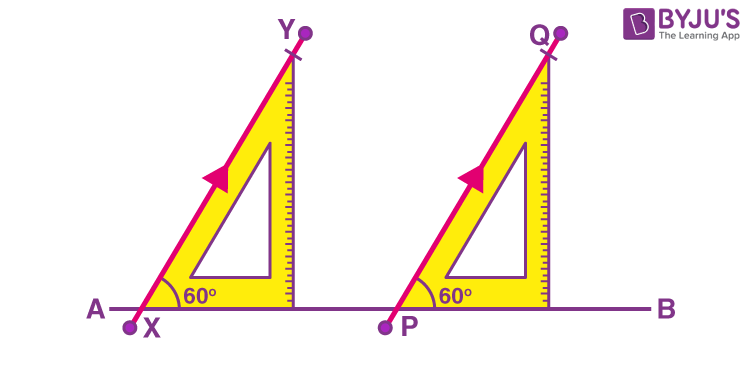He claimed that XY and PQ are parallel. Can you tell how? It’s simply right. The line segment AB serves as the transversal to XY and PQ, and angle X and angle Y are corresponding angles in this case which are in equal measure. Therefore, XY and PQ are parallel line segments.

### Solved Example

Question:

Observe the figure given below: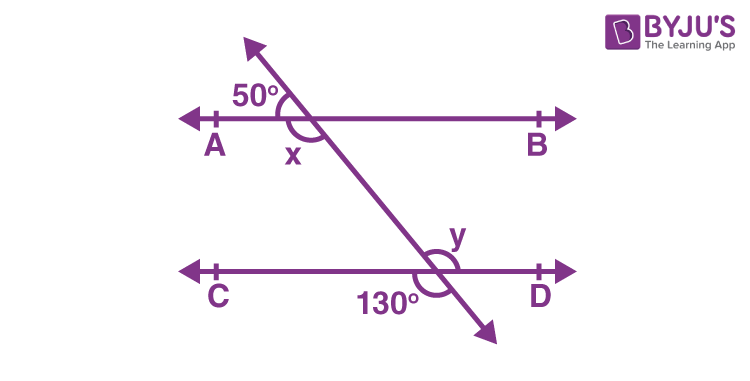Find the missing angles.

Solution:

In the given figure, AB and CD are parallel lines and these are cut by a transversal line at two different points.

Here, x and 130° are the pair of corresponding angles.

So, x = 130°

Also, y and 130° are vertically opposite angles.

Thus, y = 130°